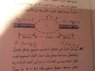# Pipe Radius: Calculate Diameter of Middle Part

• vinamas

## Homework Statement

The following image shows the parts A and B of a pipe where the middle part goes under a hill the length of both parts A and B is 30m and the diameter of them each is 2cm where as the middle part has a length of 110m to determine the middle part's diameter one engineer decided to push water in the pipe with a speed of 2.50 m\s in part A he dropped a small coin in the flow and noticed that it took 88.8 seconds for it to start from A and exit from B.
1)calculate the diameter of the middle pipe

## Homework Equations

v=d\s
A1*v1=A2*v2. (Continuity equation)
A=pi*r^2[/B]

## The Attempt at a Solution

Finding the area of a and b:
0.02^2*pi=0.00125m^2
Finding the time it would take to travel through A and B (not including mid pipe)
2.50=30/s
s=12
So for a and b it would take 24 seconds
For mid part it would take 88.8-24=64.8
Mid part speed
110/64.8=1.697m\s
So
0.00125*2.50=1.697*A2
Area=0.001841
0.0018141=pi*r^2
R=0.024m
Answer sheet says that the diameter is 3.60cm
help!

#### Attachments

•image.jpg
38.6 KB · Views: 406
Finding the area of a and b:
0.02^2*pi=0.00125m^2
... the diameter of the pipe is 2cm.

Note: you do not have to compute the area ...
You can express: ##A_1v_1 = A_2v_2## in terms of the two diameters ##d_1## and ##d_2## using ##A=\pi d^2/4##.

Now I've had a chance to check.
Assuming the coin travels at the same (average) speed as the water - it travels 60m at u=2.5m/s, this takes 60/2.5=24s
Total travel time is 88.8s to time through the entire length, so the time spent in the middle section is 88.8-24=64.8s
This gives an average speed through that section as v=110/64.8 = 1.6975m/s = 169.75cm/s
Volume flow rate is a constant for incompressible flow: we want to find hidden diameter d, given revealed diameter, u, and v
##\pi (d^2/4)v = \pi (2^2/4)u \implies d = 2\sqrt{2.5/1.6975} = 2.43##cm (2dp)
... in agreement with your answer. (notice I did not need to convert the units of diameter to meters.)

This suggests the model answer is incorrect, or uses a different model.
You can work out what the time through the entire pipe would be if the middle section were 3.6cm in diameter.

Now I've had a chance to check.
Assuming the coin travels at the same (average) speed as the water - it travels 60m at u=2.5m/s, this takes 60/2.5=24s
Total travel time is 88.8s to time through the entire length, so the time spent in the middle section is 88.8-24=64.8s
This gives an average speed through that section as v=110/64.8 = 1.6975m/s = 169.75cm/s
Volume flow rate is a constant for incompressible flow: we want to find hidden diameter d, given revealed diameter, u, and v
##\pi (d^2/4)v = \pi (2^2/4)u \implies d = 2\sqrt{2.5/1.6975} = 2.43##cm (2dp)
... in agreement with your answer. (notice I did not need to convert the units of diameter to meters.)